# Biconditional Statements 2 4 and Definitions Objective Write

• Slides: 15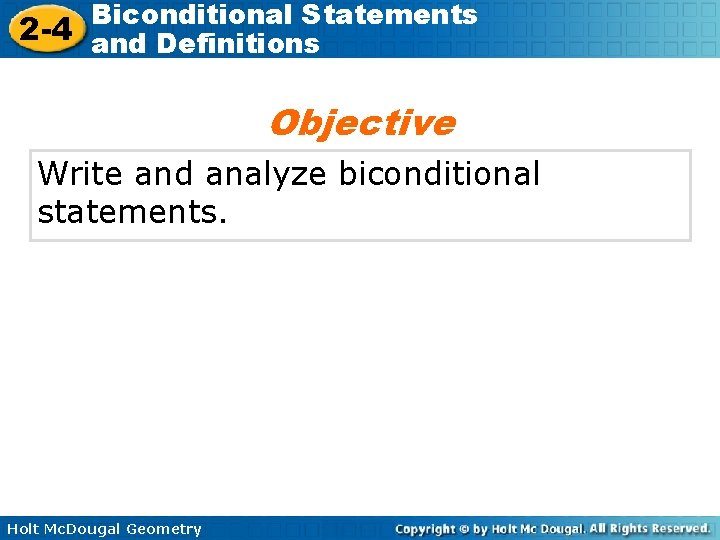Biconditional Statements 2 -4 and Definitions Objective Write and analyze biconditional statements. Holt Mc. Dougal Geometry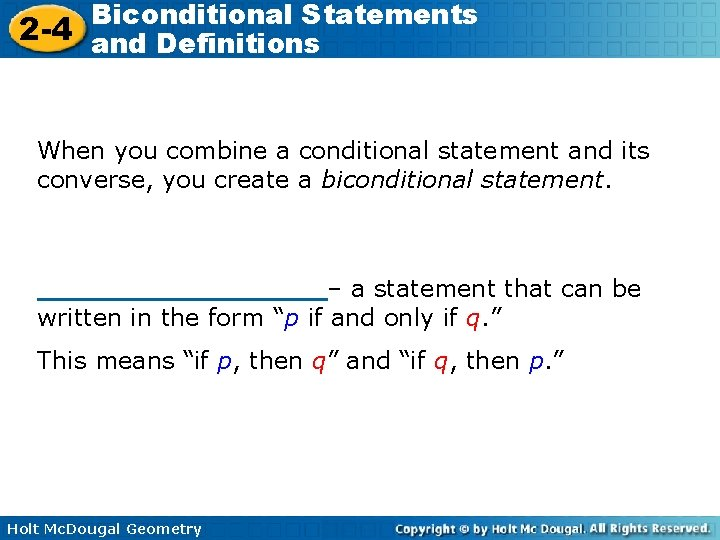Biconditional Statements 2 -4 and Definitions When you combine a conditional statement and its converse, you create a biconditional statement. _________– a statement that can be written in the form “p if and only if q. ” This means “if p, then q” and “if q, then p. ” Holt Mc. Dougal Geometry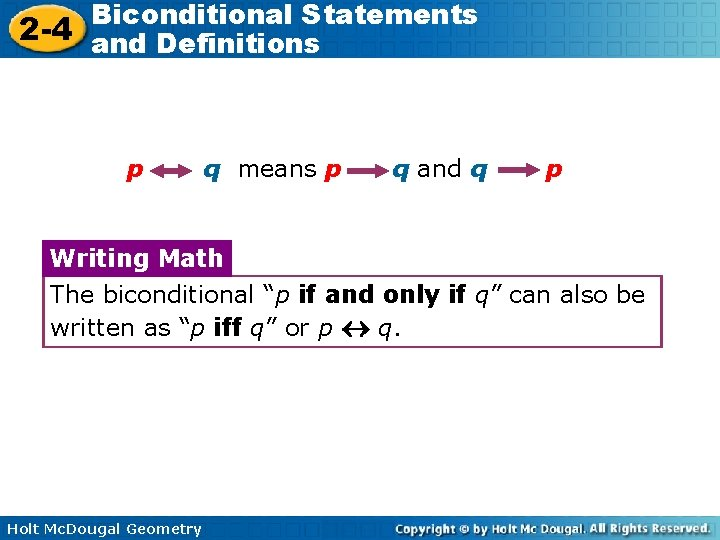Biconditional Statements 2 -4 and Definitions p q means p q and q p Writing Math The biconditional “p if and only if q” can also be written as “p iff q” or p q. Holt Mc. Dougal GeometryBiconditional Statements 2 -4 and Definitions Example 1 a Write the conditional statement and converse within the biconditional. An angle is acute iff its measure is greater than 0° and less than 90°. Let x and y represent the following. x: An angle is acute. y: An angle has a measure that is greater than 0 and less than 90. Holt Mc. Dougal Geometry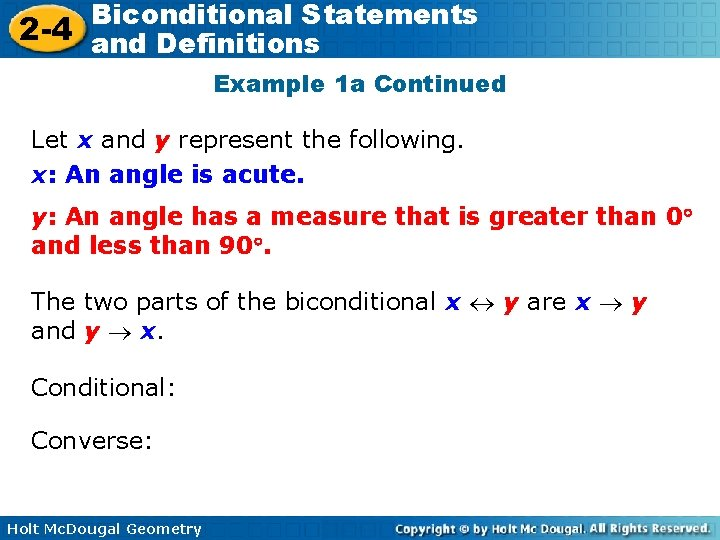Biconditional Statements 2 -4 and Definitions Example 1 a Continued Let x and y represent the following. x: An angle is acute. y: An angle has a measure that is greater than 0 and less than 90. The two parts of the biconditional x y are x y and y x. Conditional: Converse: Holt Mc. Dougal GeometryBiconditional Statements 2 -4 and Definitions Example 1 b Write the conditional statement and converse within the biconditional. Cho is a member if and only if he has paid the \$5 dues. Holt Mc. Dougal Geometry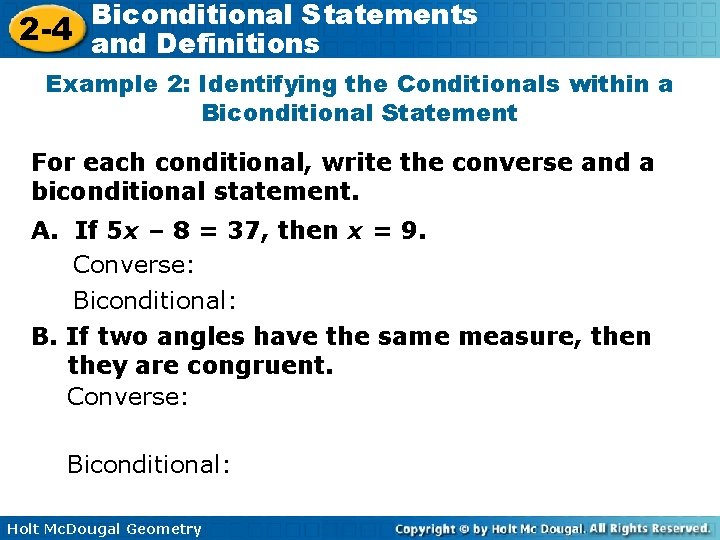Biconditional Statements 2 -4 and Definitions Example 2: Identifying the Conditionals within a Biconditional Statement For each conditional, write the converse and a biconditional statement. A. If 5 x – 8 = 37, then x = 9. Converse: Biconditional: B. If two angles have the same measure, then they are congruent. Converse: Biconditional: Holt Mc. Dougal Geometry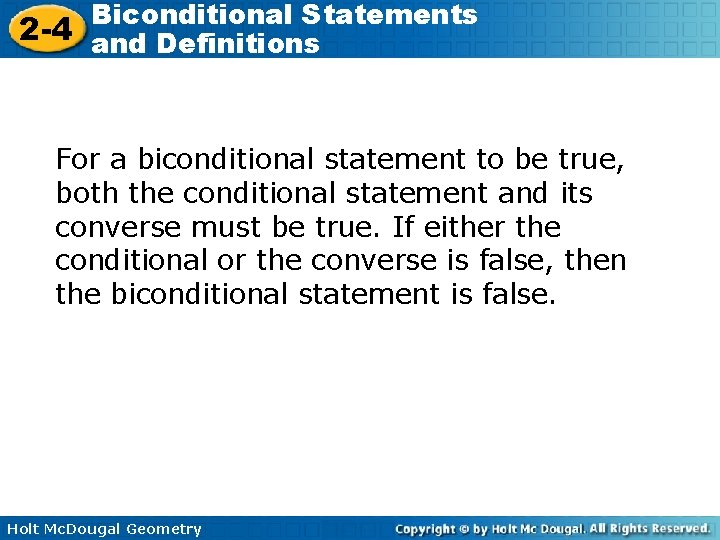Biconditional Statements 2 -4 and Definitions For a biconditional statement to be true, both the conditional statement and its converse must be true. If either the conditional or the converse is false, then the biconditional statement is false. Holt Mc. Dougal GeometryBiconditional Statements 2 -4 and Definitions Example 3 A: Analyzing the Truth Value of a Biconditional Statement Determine if the biconditional is true. If false, give a counterexample. A rectangle has side lengths of 12 cm and 25 cm if and only if its area is 300 cm 2. Holt Mc. Dougal Geometry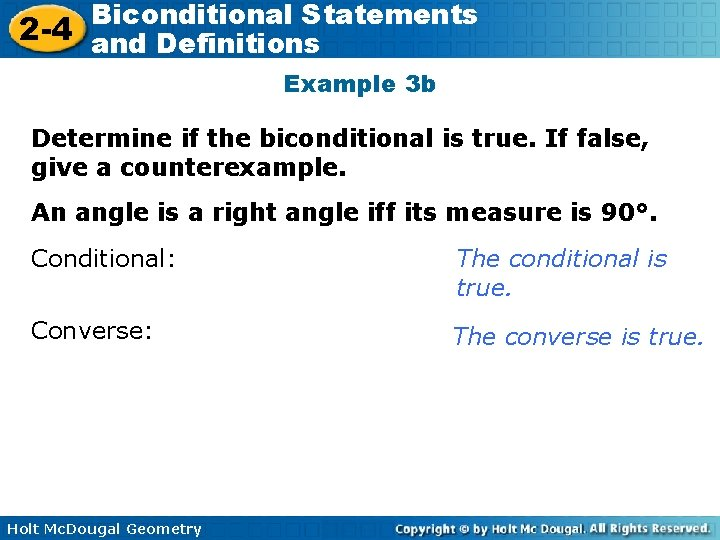Biconditional Statements 2 -4 and Definitions Example 3 b Determine if the biconditional is true. If false, give a counterexample. An angle is a right angle iff its measure is 90°. Conditional: The conditional is true. Converse: The converse is true. Holt Mc. Dougal Geometry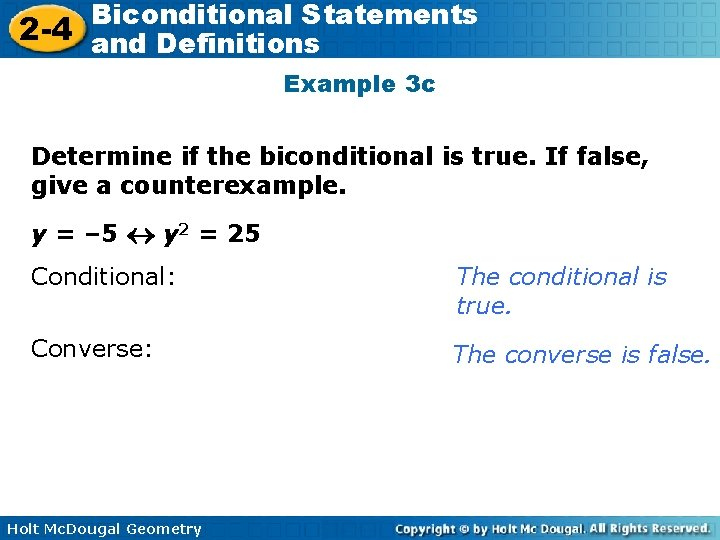Biconditional Statements 2 -4 and Definitions Example 3 c Determine if the biconditional is true. If false, give a counterexample. y = – 5 y 2 = 25 Conditional: The conditional is true. Converse: The converse is false. Holt Mc. Dougal Geometry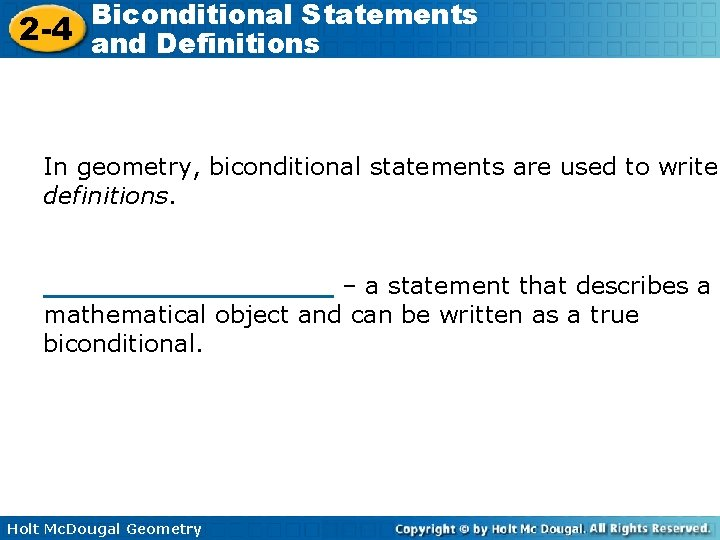Biconditional Statements 2 -4 and Definitions In geometry, biconditional statements are used to write definitions. _________ – a statement that describes a mathematical object and can be written as a true biconditional. Holt Mc. Dougal GeometryBiconditional Statements 2 -4 and Definitions _________ – a closed plane figure formed by three or more line segments. Holt Mc. Dougal GeometryBiconditional Statements 2 -4 and Definitions _________ – three-sided polygon _________– four-sided polygon. Holt Mc. Dougal Geometry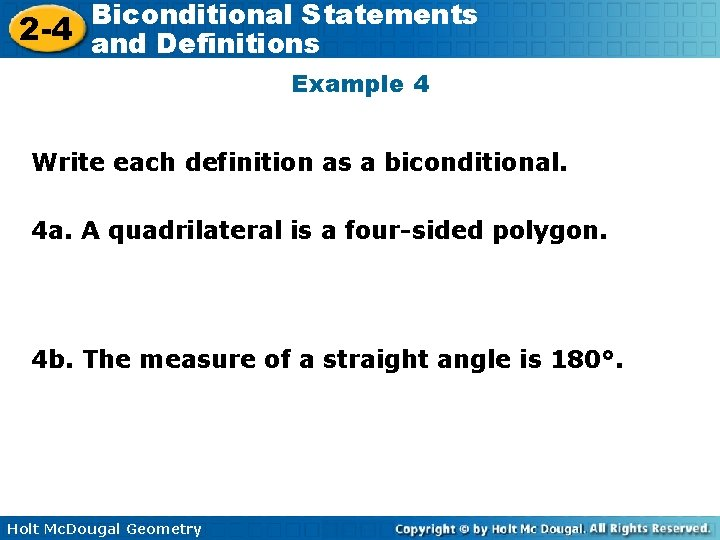Biconditional Statements 2 -4 and Definitions Example 4 Write each definition as a biconditional. 4 a. A quadrilateral is a four-sided polygon. 4 b. The measure of a straight angle is 180°. Holt Mc. Dougal Geometry